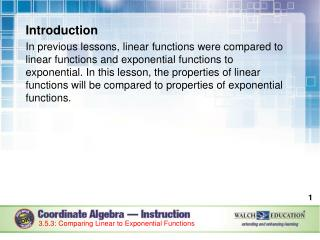DownloadDownload PresentationIntroduction

# Introduction

Download Presentation## Introduction

- - - - - - - - - - - - - - - - - - - - - - - - - - - E N D - - - - - - - - - - - - - - - - - - - - - - - - - - -
##### Presentation Transcript

1. Introduction In previous lessons, linear functions were compared to linear functions and exponential functions to exponential. In this lesson, the properties of linear functions will be compared to properties of exponential functions. 3.5.3: Comparing Linear to Exponential Functions

2. Key Concepts Linear functions are written in the form f(x) = mx + b. Afactor is one of two or more numbers or expressions that when multiplied produce a given product. The variable of a linear function is a factor of the function. As the value of x increases, the value of f(x) will increase at a constant rate. 3.5.3: Comparing Linear to Exponential Functions

3. Key Concepts, continued The rate of change of linear functions remains constant. Exponential functions are written in the form g(x) = abx. The variable of an exponential function is part of the exponent. As the value of x increases, the value of g(x) will increase by a multiple of b. 3.5.3: Comparing Linear to Exponential Functions

4. Key Concepts, continued As discussed previously, the rate of change of an exponential function varies depending on the interval observed. Graphs of exponential functions of the form g(x) = abx, where b is greater than 1, will increase faster than graphs of linear functions of the form f(x) = mx + b. A quantity that increases exponentially will always eventually exceed a quantity that increases linearly. 3.5.3: Comparing Linear to Exponential Functions

5. Common Errors/Misconceptions incorrectly determining the rate of change assuming that a positive slope will be steeper than a negative slope interchanging the x- and y-intercepts assuming the rate of change of a function is linear by only referencing one interval 3.5.3: Comparing Linear to Exponential Functions

6. Guided Practice Example 2 At approximately what point does the value of f(x) exceed the value of g(x) if and g(x) = 0.5x? Justify your answer with a graph. 3.5.3: Comparing Linear to Exponential Functions

7. Guided Practice: Example 2, continued Make a general observation. is an exponential function of the form g(x) = abx. The variable x is the exponent. g(x) = 0.5x is a linear function of the form f(x) = mx + b. The variable x is multiplied by the coefficient 0.5. 3.5.3: Comparing Linear to Exponential Functions

8. Guided Practice: Example 2, continued Create a table of values. Substitute values for x into each function. 3.5.3: Comparing Linear to Exponential Functions

9. Guided Practice: Example 2, continued Graph both functions on the same coordinate plane. Use the tables of values created in order to plot both functions. 3.5.3: Comparing Linear to Exponential Functions

10. Guided Practice: Example 2, continued 3.5.3: Comparing Linear to Exponential Functions

11. Guided Practice: Example 2, continued Identify the approximate point where f(x) is greater than g(x). It can be seen from the graph that both functions are equal where xis approximately equal to 28. When x is greater than 28, f(x) is greater than g(x). ✔ 3.5.3: Comparing Linear to Exponential Functions

12. Guided Practice: Example 2, continued 12 3.5.3: Comparing Linear to Exponential Functions

13. Guided Practice Example 3 Lena has been offered a job with two salary options. The first option is modeled by the function f(x) = 500x + 31,000, where f(x) is her salary in dollars after x years. The second option is represented by the function g(x) = 29,000(1.04) x, where g(x) is her salary in dollars after x years. If Lena is hoping to keep this position for at least 5 years, which salary option should she choose? Support your answer with a graph. 3.5.3: Comparing Linear to Exponential Functions

14. Guided Practice: Example 3, continued Make a general observation. f(x) = 500x + 31,000 is a linear function of the form f(x) = mx + b. The variable x is multiplied by the coefficient 500 and added to the constant 31,000. g(x) = 29,000(1.04) x is an exponential function of the form g(x) = abx. The variable x is the exponent. 3.5.3: Comparing Linear to Exponential Functions

15. Guided Practice: Example 3, continued Create a table of values. Substitute values for x into each function. 3.5.3: Comparing Linear to Exponential Functions

16. Guided Practice: Example 3, continued Graph both functions on the same coordinate plane. Use the tables of values created in order to plot both functions. 3.5.3: Comparing Linear to Exponential Functions

17. Guided Practice: Example 3, continued Yearly salary (in thousands of dollars) Years 3.5.3: Comparing Linear to Exponential Functions

18. Guided Practice: Example 3, continued Identify the approximate point where g(x) is greater than f(x). It can be seen from the graph that after 3 years, g(x) is greater than f(x). If Lena is hoping to keep this position for at least 5 years, it is in her best interest to choose the salary option modeled by g(x) = 29,000(1.04)x. ✔ 3.5.3: Comparing Linear to Exponential Functions

19. Guided Practice: Example 3, continued 19 3.5.3: Comparing Linear to Exponential Functions